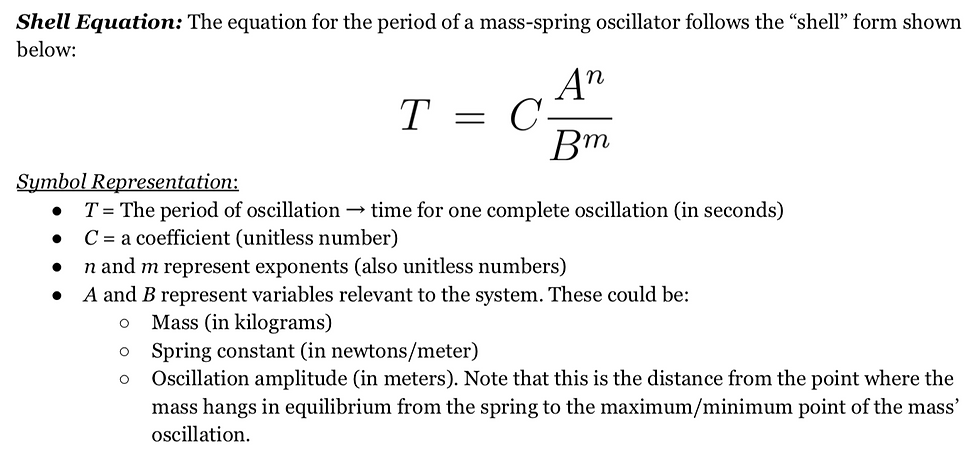top of pageSearch
• Maxwell Fazio

# Using “Shell Equations” to Scaffold Inquiry Labs

Updated: Jun 9, 2022

A practice for guiding the inquiry process in the development of accurate mathematical models.Inquiry vs. Content

Are we supposed to use inquiry or are we supposed to teach content? Sometimes the two things go hand in hand, but we've all seen that open inquiry, while valuable, takes a long time and often results in students coming to understandings that are not grounded in the content that we are hoping for them to learn. Sometimes this is fine, but we work in the real world and have time constraints as well as content that we are responsible for teaching.

Motivating a lab by introducing a "shell equation" constrains the inquiry process without telling students what to do.

Inquiry of course, has benefits! Students practice real science skills and construct their own understandings and mathematical models. However, we often hope that students will draw deep conclusions from their inquiry that give them insights into the content that they wouldn’t get without the process of discovery. Motivating a lab by introducing a shell equation constrains the inquiry process without telling students what to do. Instead of telling them what methods to follow, it gives them a basis of knowledge to build upon. It gives them the freedom and the responsibility to make the necessary methodological decisions.

So What is a Shell Equation?

A shell equation is an incomplete mathematical model. Take for example the equation for the rotational inertia of a point mass. It is shown below along with what could be considered its shell form.Rotational Inertia EquationShell Form of the Equation

The shell form gives framework for a mathematical model to be built upon. In the example above A = m, X = 1, B = r, and Y = 2. The purpose of the shell equation is to give students a sufficient starting point from which to design their own inquiry. The goal for them is to collect data and use their data to make evidence-based claims about what the actual mathematical model should be. In other words, to "fill in" the shell equation.

Do we have time for this? Does this kind of constrained inquiry build skills that are relevant for APP1 and APP2?

Just take a look at these released FRQs:

All of them require students to evaluate the validity of mathematical models that are not explicitly taught in APP1. The reasoning required in these questions is very similar to developing and refining mathematical models within shell equation constraints.

First Implementation: Terminal Velocity of Coffee FiltersLab Setup for Terminal Velocity of Coffee Filters

The tricky part is in figuring out what systems to use and how to write the shell equations so that students have just enough information so that with smart inquiry choices they can correctly determine the correct mathematical model. Early in the year we did the classic terminal velocity with coffee filters lab, but I was very careful in how I chose to frame their objective. This was before I came up with the snappy name "shell equation."

The Experiment: I explicitly told students to take the mass of the coffee filter as their independent variable and to measure its terminal velocity as their dependent variable. Students manipulated mass of coffee filters by stacking multiple filters together. Then I gave them the directions below to build their analysis upon. (Access the full student document on my classroom resources page).

Students were able to find the power of the function by looking at their graphs. Most of my students used logger pro and fit a power function (y=Ax^B) to see what power the computer used to fit their data. Students then used logical reasoning to place the other variables in the numerator or denominator of the equation.A More Polished Approach: Period of a Mass-Spring Oscillator

More recently when we came to simple harmonic motion, I chose to take a similar approach. My goal was for students to construct the mathematical model for the period of a mass spring oscillator, giving them as much freedom as possible to make choices in the inquiry process. I gave them the following shell equation:Shell Equation for Mass-Spring Oscillator

Students were asked to design and conduct whatever experiments they would need in order to complete the mathematical model. They were then expected to argue their mathematical model using their data as evidence. (Again, the full student task sheet is available on my resources page.)

The discussions surrounding their experimental designs were so rich! Most groups recognized that right away they should try three experiments (Period vs. Mass, Period vs. Amplitude, and Period vs. Spring Constant) and then use those to figure out which variables are in the equation and what the exponents are. (Note that I have spring sets with known spring constants so students didn't have to take time to calculate this for every spring.)

Some groups struggled to design a method for finding the coefficient "C", but with a bit of coaching all groups came to understand that once they had the variables figured out, they could go back to their graphs and then use the coefficients generated by their autofit functions to find it.

Student Exemplar: I'm including a very strong student sample to illustrate what I was striving for them to achieve.bottom of page